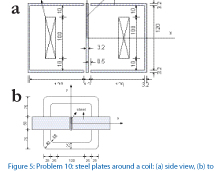Peng Chen with Advisors J.M. Jin and P.T. KreinAccurate nonlinear simulations of electric machines are increasingly important for optimizing system arrangements. The goal of this work is to apply the time-domain finite element method (TDFEM) to simulate the three-dimensional (3-D) nonlinear machine problem. The performance of the TDFEM method was first investigated using a benchmark problem for the calculation of eddy currents within a 3D linear structure. The good agreement of calculated magnetic flux with measured results demonstrates the validity of the formulation and algorithm for solving the 3-D linear eddy current problem.

The formulation was extended to address nonlinear materials, which are particularly crucial for accurate machine simulations. The TEAM benchmark problem 10, where three steel plates composed of nonlinear materials surround a coil, is used. The side and top view of the simulated model are shown in Figure 5(a) and (b), respectively. In this model, the reluctivity of the nonlinear material (steel plates) depends on the strength of the magnetic
flux density and their relation is characterized through curves obtained by using cubic spline interpolation of the measured data.
The resulting system of nonlinear equations is solved using the Newton-Raphson iterative method in each time step. More specifically, with a proper initial guess at the beginning of the time-marching scheme, a set of nonlinear equations is solved iteratively. The converged solution obtained from the current time-step is then applied as the new initial guess for the iteration in the next time-step. This process is repeated throughout the entire range of interest. This example is being tested and the results are compared to verify the formulation and algorithm.

This research is supported by the Grainger Center for Electric Machinery and Electromechanics.